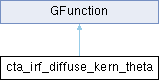GammaLib  1.7.0.dev
cta_irf_diffuse_kern_theta Class Reference

Kernel for IRF offest angle integration of the diffuse source model. More...

#include <GCTAResponse_helpers.hpp>

Inheritance diagram for cta_irf_diffuse_kern_theta:## Public Member Functions

cta_irf_diffuse_kern_theta (const GCTAResponseIrf *rsp, const GModelSpatial *model, const GMatrix *rot, const double &theta, const double &phi, const double &zenith, const double &azimuth, const GEnergy &srcEng, const GTime &srcTime, const double &srcLogEng, const GEnergy &obsEng, const double &eta, const int &min_iter, const int &max_iter, const double &resolution)

double eval (const double &theta)
Kernel for IRF offest angle integration of the diffuse source model. More...Public Member Functions inherited from GFunction
GFunction (void)
Void constructor. More...

GFunction (const GFunction &function)
Copy constructor. More...

virtual ~GFunction (void)
Destructor. More...

GFunctionoperator= (const GFunction &function)
Assignment operator. More...

## Protected Attributes

const GCTAResponseIrfm_rsp
CTA response. More...

const GModelSpatialm_model
Spatial model. More...

const GMatrixm_rot
Rotation matrix. More...

double m_theta
Photon offset angle. More...

double m_phi
Photon azimuth angle. More...

double m_zenith
Pointing zenith angle. More...

double m_azimuth
Pointing azimuth angle. More...

GEnergy m_srcEng
True photon energy. More...

GTime m_srcTime
True photon arrival time. More...

double m_srcLogEng
True photon log energy. More...

GEnergy m_obsEng
Measured event energy. More...

double m_sin_eta
Sine of angular distance between. More...

double m_cos_eta
Cosine of angular distance between. More...

int m_min_iter

int m_max_iter

double m_resolutionProtected Member Functions inherited from GFunction
void init_members (void)
Initialise class members. More...

void copy_members (const GFunction &function)
Copy class members. More...

void free_members (void)
Delete class members. More...

## Detailed Description

Kernel for IRF offest angle integration of the diffuse source model.

This class implements the integration kernel $$K(\theta)$$ for the integration

$\int_{0}^{\theta_{\rm max}} K(\theta | E, t) d\theta$

of diffuse models. The eval() method computes

$K(\theta | E, t) = \sin \theta \times PSF(\theta) \int_{0}^{2\pi} S_{\rm p}(\theta, \phi | E, t) \, Aeff(\theta, \phi) \, Edisp(\theta, \phi) d\phi$

where

• $$S_{\rm p}(\theta, \phi | E, t)$$ is the diffuse model,
• $$PSF(\theta)$$ is the azimuthally symmetric Point Spread Function,
• $$Aeff(\theta, \phi)$$ is the effective area,
• $$Edisp(\theta, \phi)$$ is the energy dispersion,
• $$\theta$$ is the distance from the PSF centre, and
• $$\phi$$ is the azimuth angle.

Definition at line 771 of file GCTAResponse_helpers.hpp.

## Constructor & Destructor Documentation

 cta_irf_diffuse_kern_theta::cta_irf_diffuse_kern_theta ( const GCTAResponseIrf * rsp, const GModelSpatial * model, const GMatrix * rot, const double & theta, const double & phi, const double & zenith, const double & azimuth, const GEnergy & srcEng, const GTime & srcTime, const double & srcLogEng, const GEnergy & obsEng, const double & eta, const int & min_iter, const int & max_iter, const double & resolution )
inline

Definition at line 773 of file GCTAResponse_helpers.hpp.

## Member Function Documentation

 double cta_irf_diffuse_kern_theta::eval ( const double & theta )
virtual

Kernel for IRF offest angle integration of the diffuse source model.

Parameters
 [in] theta Offset angle with respect to observed photon direction (radians).

Computes

$K(\theta | E, t) = \sin \theta \times PSF(\theta) \int_{0}^{2\pi} S_{\rm p}(\theta, \phi | E, t) \, Aeff(\theta, \phi) \, Edisp(\theta, \phi) d\phi$

The PSF is assumed to be azimuthally symmetric, hence the PSF is computed outside the azimuthal integration.

Note that the integration is only performed for $$\theta>0$$. Otherwise zero is returned.

Implements GFunction.

Definition at line 1287 of file GCTAResponse_helpers.cpp.

## Member Data Documentation

 double cta_irf_diffuse_kern_theta::m_azimuth
protected

Pointing azimuth angle.

Definition at line 812 of file GCTAResponse_helpers.hpp.

Referenced by eval().

 double cta_irf_diffuse_kern_theta::m_cos_eta
protected

Cosine of angular distance between.

Definition at line 820 of file GCTAResponse_helpers.hpp.

Referenced by eval().

 int cta_irf_diffuse_kern_theta::m_max_iter
protected

Definition at line 824 of file GCTAResponse_helpers.hpp.

Referenced by eval().

 int cta_irf_diffuse_kern_theta::m_min_iter
protected

Definition at line 823 of file GCTAResponse_helpers.hpp.

Referenced by eval().

 const GModelSpatial* cta_irf_diffuse_kern_theta::m_model
protected

Spatial model.

Definition at line 807 of file GCTAResponse_helpers.hpp.

Referenced by eval().

 GEnergy cta_irf_diffuse_kern_theta::m_obsEng
protected

Measured event energy.

Definition at line 816 of file GCTAResponse_helpers.hpp.

Referenced by eval().

 double cta_irf_diffuse_kern_theta::m_phi
protected

Photon azimuth angle.

Definition at line 810 of file GCTAResponse_helpers.hpp.

Referenced by eval().

 double cta_irf_diffuse_kern_theta::m_resolution
protected

Definition at line 825 of file GCTAResponse_helpers.hpp.

Referenced by eval().

 const GMatrix* cta_irf_diffuse_kern_theta::m_rot
protected

Rotation matrix.

Definition at line 808 of file GCTAResponse_helpers.hpp.

Referenced by eval().

 const GCTAResponseIrf* cta_irf_diffuse_kern_theta::m_rsp
protected

CTA response.

Definition at line 806 of file GCTAResponse_helpers.hpp.

Referenced by eval().

 double cta_irf_diffuse_kern_theta::m_sin_eta
protected

Sine of angular distance between.

Definition at line 817 of file GCTAResponse_helpers.hpp.

Referenced by eval().

 GEnergy cta_irf_diffuse_kern_theta::m_srcEng
protected

True photon energy.

Definition at line 813 of file GCTAResponse_helpers.hpp.

Referenced by eval().

 double cta_irf_diffuse_kern_theta::m_srcLogEng
protected

True photon log energy.

Definition at line 815 of file GCTAResponse_helpers.hpp.

Referenced by eval().

 GTime cta_irf_diffuse_kern_theta::m_srcTime
protected

True photon arrival time.

Definition at line 814 of file GCTAResponse_helpers.hpp.

Referenced by eval().

 double cta_irf_diffuse_kern_theta::m_theta
protected

Photon offset angle.

Definition at line 809 of file GCTAResponse_helpers.hpp.

Referenced by eval().

 double cta_irf_diffuse_kern_theta::m_zenith
protected

Pointing zenith angle.

Definition at line 811 of file GCTAResponse_helpers.hpp.

Referenced by eval().

The documentation for this class was generated from the following files: